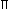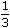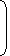# Volume And Surface Area- Simple Formulae##### VOLUME AND SURFACE AREA
1. CUBOIDLet length = l, breadth = b and height = h units. Then
1. Volume = (l x b x h) cubic units.
2. Surface area = 2(lb + bh + lh) sq. units.
3. Diagonal = l2 + b2 + h2 units.
2. CUBELet each edge of a cube be of length a. Then,
1. Volume = a3 cubic units.
2. Surface area = 6a2 sq. units.
3. Diagonal = 3a units.
3. CYLINDERLet radius of base = r and Height (or length) = h. Then,
1. Volume = (r2h) cubic units.
2. Curved surface area = (2rh) sq. units.
3. Total surface area = 2r(h + r) sq. units.
4. CONELet radius of base = r and Height = h. Then,
1. Slant height, l = h2 + r2 units.
2. Volume =r2hcubic units.
3. Curved surface area = (rl) sq. units.
4. Total surface area = (rl +r2) sq. units.
5. SPHERELet the radius of the sphere be r. Then,
1. Volume =r3cubic units.
2. Surface area = (4r2) sq. units.
6. HEMISPHERELet the radius of a hemisphere be r. Then,
1. Volume =r3cubic units.
2. Curved surface area = (2r2) sq. units.
3. Total surface area = (3r2) sq. units.Note: 1 litre = 1000 cm3.

#### A right triangle with sides 3 cm, 4 cm and 5 cm is rotated the side of 3 cm to form a cone. The volume of the cone so formed is:

Correct! Wrong!

#### The slant height of a right circular cone is 10 m and its height is 8 m. Find the area of its curved surface.

Correct! Wrong!

#### A metallic sheet is of rectangular shape with dimensions 48 m x 36 m. From each of its corners, a square is cut off so as to make an open box. If the length of the square is 8 m, the volume of the box (in m3) is:

Correct! Wrong!

#### The curved surface area of a cylindrical pillar is 264 m2 and its volume is 924 m3. Find the ratio of its diameter to its height.

Correct! Wrong!

#### 15. How many bricks, each measuring 25 cm x 11.25 cm x 6 cm, will be needed to build a wall of 8 m x 6 m x 22.5 cm?

Correct! Wrong!

Volume and Surface area
Wonder performance...
Great.....
Good....
Work hard...